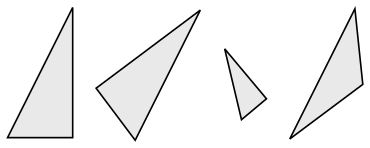# Congruence (geometry) facts for kids

Kids Encyclopedia FactsAn example of congruence. The two triangles on the left are congruent, while the third is similar to them. The last triangle is neither similar nor congruent to any of the others. Note that congruence permits alteration of some properties, such as location and orientation, but leaves others unchanged, like distance and angles. The unchanged properties are called invariants.

In geometry, two figures or objects are congruent if they have the same shape and size. Also if one has the same shape and size as the mirror image of the other.

More formally, two sets of points are called congruent if, and only if, one can be transformed into the other by isometry. For isometry, rigid motions are used.

This means that one object can be repositioned and reflected (but not resized) so it coincides exactly with the other object. So two distinct plane figures on a piece of paper are congruent if we can cut them out and then match them up completely. Turning the paper over is permitted.

Congruent polygons are polygons that if you fold a regular polygon in half that is a congruent polygon.

## Images for kidsCongruence (geometry) Facts for Kids. Kiddle Encyclopedia.